# Multiple Choice Questions on Capacitors and capacitance

Question 1
Capacitance of a parallel plate air capacitor depends on
1. Thickness of conducting plates
2. Charge on the conducting plates
3. Area of the conducting plates
4. Distance of separation between the conducting plates

Question 2
Equivalent capacitance of system of capacitors shown below in the figure is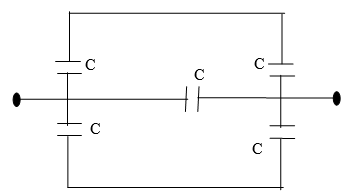1. C/2
2. 2C
3. C
4. None of these

Question 3
A parallel plate air capacitor with no dielectric between the plates is connected to the constant voltage source. How would capacitance and charge change if dielectric of dielectric constant K=2 is inserted between the plates. C0 and Q0 are the capacitance and charge of the capacitor before the introduction of the dielectric.
1. C=C0/2 ; Q=2Q0
2. C=2C0 ; Q=Q0/2
3. C=C0/2 ; Q=Q0/2
4. C=2C0 ; Q=2Q0

Question 4
A parallel plate capacitor of capacitance C is connected to a battery and is charged to a potential difference V. Another capacitor of capacitance 2C is similarly charged to a potential difference 2V. The charging battery is then disconnected and the capacitors are connected in parallel to each other in such a way that the positive terminal of one is connected to the negative terminal of the other. The final energy of the configuration is
1. Zero
2. 3CV2/2
3. 25CV2/6
4. 9CV2/2

Question 5
A parallel plate air capacitor is connected to a battery. The quantities charge, voltage, electric field, and energy associated with this capacitor are given by Q0, V0, E0 and U0 respectively. A dielectric slab is now introduced to fill the space between the plates with battery still in connection. The corresponding quantities are now given by Q, V, E and U are related to previous quantities as
1. Q> Q0
2. V> V0
3. E> E0
4. U> U0

Question 6
The effective capacitance of a capacitor is reduced when capacitors are connected in
1. series
2. parallel
3. series-parallel combination
4. none of the above

Question 7
Two identical conducting plates of plate area A are separated by a distance d to form a parallel plate air capacitor. Now a metal sheet of thickness d/2 is inserted between the plates of the capacitor. The ratio of capacitance before the insertion of plate and after the insertion of plate is
1. 1:2
2. 1:1
3. 1:2
4. √2:1

Question 8
The plates of a parallel plate air capacitor are charged to 100 V. A 2mm plate is inserted between the plates of the capacitor. To maintain the same potential difference , the distance between the capacitor plates is increased by 1.6mm. The dielectric constant of the plate is
1. 1.25
2. 5
3. 2.5
4. 4

Question 9
Four capacitors are connected as shown below in the figure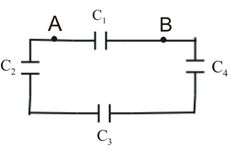Here C1=2μF , C2=3μF , C3=4μF and C4=5μF. The equivalent capacitance between A and B is
1. 1.245 μF
2. 4.446 μF
3. 9 μF
4. 3.27 μF

Question 10
Consider the figure and value of capacitances given in the Question 9 if a 4Volt battery is connected between points A and B total charge stored on the capacitors would be
1. 13.8μC
2.  10 μC
3. 22 μC
4. 4 μC

Question 11
In an isolated parallel plate capacitor of capacitance C, the four surface have charges $Q_1$, $Q_2$, $Q_3$ and $Q_4$ as shown. The potential difference between the plates is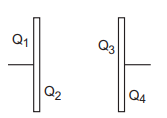(a) $\frac {Q_1 + Q_2 + Q_3 + Q_4}{2C}$
(b) $\frac {Q_2 + Q_3}{2C}$
(c) $\frac {Q_2 - Q_3}{2C}$
(d) $\frac {Q_1 + Q_4}{2C}$

Question 12
A parallel plate capacitor of capacitance C is connected to a battery and is charged to a potential difference V. Another capacitor of capacitance 2C is connected to another battery and is charged to potential difference 2V. The charging batteries are now disconnected and the capacitors are connected in parallel to each other in such a way that the positive terminal of one is connected to the negative terminal of the other. The final energy of the configuration is
(a) $\frac {3CV^2}{2}$
(b) $\frac {25CV^2}{2}$
(c) $\frac {9CV^2}{2}$
(d) zero

Question 13
A capacitor of 4 μ F is connected as shown in the circuit . The internal resistance of the battery is 0.5 Ω . The amount of charge on the capacitor plates will be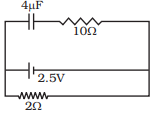(a) 0
(b) 4 μ C
(c) 16 μ C
(d) 8 μ C

Question 14
Two condensers of capacities 2C and C are joined in parallel and charged upto potential V. The battery is removed and the condenser of capacity C is filled completely with a medium of dielectric constant K. The p.d. across the capacitors will now be
(a) $\frac {3V}{K+2}$
(b) $\frac {3V}{K}$
(c) $\frac {V}{K+2}$
(d) $\frac {V}{K}$

Question 15
Five identical capacitor plates each of area A are arranged such that adjacent plates are at a distance d apart . The plates are connected to a potential difference of EMF V as shown in the figure.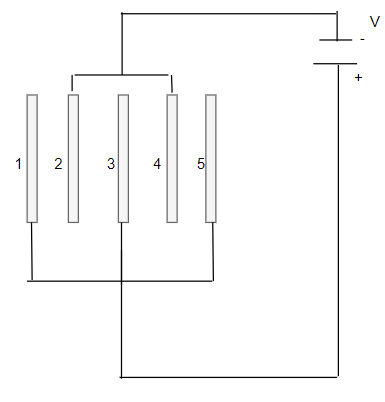The charge on plates 1 and 4 will be
(a) $\frac {\epsilon _0 A V}{d}$,$\frac {2\epsilon _0 A V}{d}$
(b) $\frac {\epsilon _0 A V}{d}$,$\frac {-2\epsilon _0 A V}{d}$
(a) $\frac {-\epsilon _0 A V}{d}$,$\frac {2\epsilon _0 A V}{d}$
(a) $\frac {\epsilon _0 A V}{d}$,$\frac {-\epsilon _0 A V}{d}$

Question 16
A parallel plate capacitor is connected to a battery as shown below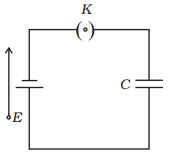Consider two situations:
A: Key K is kept closed and plates of capacitors are moved apart using insulating handle.
B: Key K is opened and plates of capacitors are moved apart using insulating handle.
Choose the correct option(s).
(a) In A : Q remains same but C changes.
(b) In B : V remains same but C changes.
(c) In A : V remains same and hence Q changes.
(d) In B : Q remains same and hence V changes.